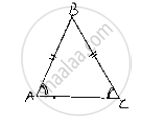# Fill the Blank in the Following So that the Following Statement is True. in a δAbc If ∠A = ∠C , Then Ab = ...... - Mathematics

Fill in the Blanks

Fill the blank in the following so that the following statement is true.

In a ΔABC if ∠A = ∠C , then AB = ......

#### Solution

In a ΔABC if , ∠A= ∠C then AB= BC
Reason: Since, the sides opposite to equal angles are equal, the side opposite to∠A
i.e., BC and ∠C i.e., AB are equalConcept: Properties of a Triangle
Is there an error in this question or solution?

#### APPEARS IN

RD Sharma Mathematics for Class 9
Chapter 12 Congruent Triangles
Exercise 12.5 | Q 6.4 | Page 62

Share